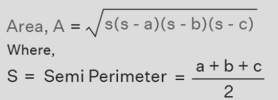# C program to calculate the area of a triangle given three sides

Here, we are going to learn how to calculate the area of a triangle given three sides using C program?
Submitted by Nidhi, on August 05, 2021

Problem Solution:

Read three edges of the triangle, and calculate the area of the triangle using the standard formula.

Formula to calculate the area of a triangle: The given sides are a, b, and c.Program:

The source code to calculate the area of a triangle given three sides is given below. The given program is compiled and executed using GCC compile on UBUNTU 18.04 OS successfully.

```// C program to calculate the area of a triangle
// given three sides

#include <stdio.h>
#include <math.h>

float calcuateAreaTriangle(int a, int b, int c)
{
float s = 0;
float area = 0;

s = (float)(a + b + c) / 2;
area = sqrt(s * (s - a) * (s - b) * (s - c));

return area;
}

int main()
{
int a = 0;
int b = 0;
int c = 0;

float area = 0;

printf("Enter the First edge of Triangle: ");
scanf("%d", &a);

printf("Enter the Second edge of Triangle: ");
scanf("%d", &b);

printf("Enter the Third edge of Triangle: ");
scanf("%d", &c);

area = calcuateAreaTriangle(a, b, c);

printf("Area of a triangle: %f\n", area);

return 0;
}
```

Output:

```RUN 1:
Enter the First edge of Triangle: 2
Enter the Second edge of Triangle: 3
Enter the Third edge of Triangle: 4
Area of a triangle: 2.904737

RUN 2:
nter the First edge of Triangle: 12
Enter the Second edge of Triangle: 10
Enter the Third edge of Triangle: 8
Area of a triangle: 39.686268

RUN 3:
Enter the First edge of Triangle: 24
Enter the Second edge of Triangle: 20
Enter the Third edge of Triangle: 16
Area of a triangle: 158.745071
```

Explanation:

In the above program, we created two functions calcuateAreaTriangle() and main(). The calcuateAreaTriangle() function is used to calculate the area of the triangle based on the given three edges of the triangle as a parameter.

In the main() function, we read the three edges of the triangle from the user. Then we called the calcuateAreaTriangle() function and got the area of the triangle and printed the result on the console screen.

What's New

Top Interview Coding Problems/Challenges!

Languages: » C » C++ » C++ STL » Java » Data Structure » C#.Net » Android » Kotlin » SQL
Web Technologies: » PHP » Python » JavaScript » CSS » Ajax » Node.js » Web programming/HTML
Solved programs: » C » C++ » DS » Java » C#
Aptitude que. & ans.: » C » C++ » Java » DBMS
Interview que. & ans.: » C » Embedded C » Java » SEO » HR
CS Subjects: » CS Basics » O.S. » Networks » DBMS » Embedded Systems » Cloud Computing
» Machine learning » CS Organizations » Linux » DOS
More: » Articles » Puzzles » News/Updates